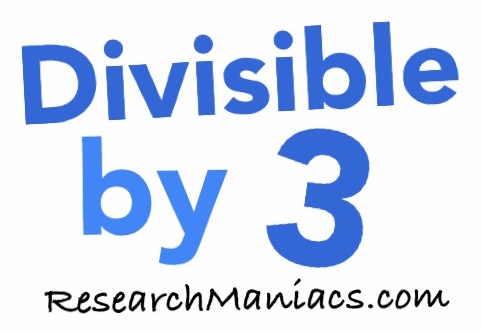Is 100 divisible by 3?

 Is 100 divisible by 3? In other words, if you divide 100 by 3, will you get a whole number with no remainder? Of course, you could use a calculator to find out if 100 is divisible by 3, but what fun would that be? To find out if 100 is divisible by 3, we will add up the digits that make 100 as follows: 1 + 0 + 0 = 1 We know that if the sum of the numbers that make up 100 is divisible by 3, then 100 is divisible by 3. Since the sum of the digits in 100 is not divisible by 3, 100 is also NOT divisible by 3. Thus, the answer to the question "Is 100 divisible by 3?" is as follows: No Note: If you divide 100 by 3, you get 33.33 which is not a whole number. Is 101 divisible by 3? Can you do it on your own now? If not, go here for the next number on our list.Divisible by 3? Do you need to check another number? Enter a number below to see if it is divisible by 3.